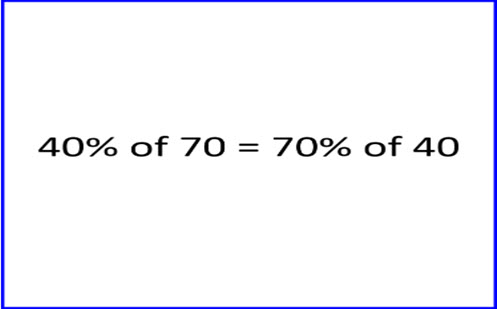Go to website

# Percentage of

This inquiry lesson is aimed to extend learning and explore students understanding of percentages as they verify; test other cases; make conjectures; and reason, generalise and prove. Students can work collaboratively to determine the answer to various 'percentage of' questions, comparing their answers to other prompts and statements. Students then identify commonalities and patterns in their answers. Many examples of students' responses to the prompt are provided for analysis. Teachers can use the inquiry as a teaching tool and to probe questions to assess student understanding.

Year level(s) Year 7
Audience Student, Teacher
Teaching strategies and pedagogical approaches Collaborative learning, Differentiated teaching, Growth mindset, Feedback, Mathematics investigation, Metacognitive strategies, Questioning, Structuring lessons, Worked examples
Keywords percentage

## Curriculum alignment

Curriculum connections ICT general capability, Numeracy
Strand and focus Algebra, Number, Build understanding, Apply understanding
Topics Fractions, Operating with number, Percentage, Decimals, Multiplication and division
AC: Mathematics (V9.0) content descriptions
AC9M7N06
Use the 4 operations with positive rational numbers including fractions, decimals and percentages to solve problems using efficient calculation strategies

Multiplicative strategies (P10)
Proportional thinking (P2)
Number and place value (P10)
Interpreting fractions (P8)